Stationarity and Autocorrelation Functions of VXX-Time Series Analysis in Python

In the previous post, we presented a system for trading VXX, a volatility Exchange Traded Note. The trading system was built based on simple moving averages.  In this post, we are going to examine the time series properties of VXX in more details.

The figure below shows the VXX and its 200-day moving average for the last 5 years. From the Figure, it’s evident that the time series has a strong downward drift. The cause of this downward drift has been discussed to a great extent in the investment community.  It will not be a subject of this post.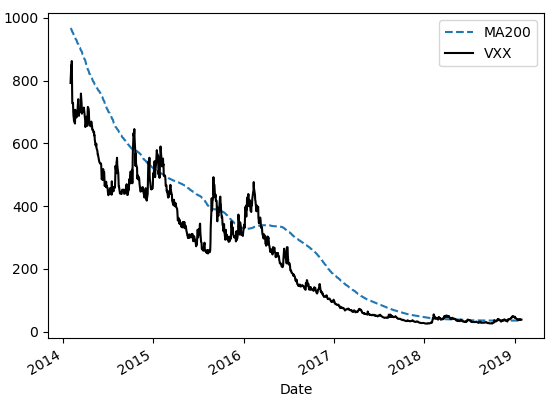Next, we calculate the daily returns of VXX and perform the Augmented Dickey-Fuller test to check the stationarity of the return series.  According to Wikipedia,

..An augmented Dickey-Fuller test (ADF) tests the null hypothesis that a unit root is present in a time series sample. The alternative hypothesis is different depending on which version of the test is used, but is usually stationarity or trend-stationarity. It is an augmented version of the Dickey-Fuller test for a larger and more complicated set of time series models. The augmented Dickey-Fuller (ADF) statistic, used in the test, is a negative number. The more negative it is, the stronger the rejection of the hypothesis that there is a unit root at some level of confidence. Read more

We utilize Python to run ADF test on the return series. The test statistic is -19.5 and the p-value is zero. Therefore the return series is stationary.

We then proceed to examine the autocorrelation functions of VXX returns.

Autocorrelation, also known as serial correlation, is the correlation of a signal with a delayed copy of itself as a function of delay. Informally, it is the similarity between observations as a function of the time lag between them. The analysis of autocorrelation is a mathematical tool for finding repeating patterns… Read more

The plot below shows the autocorrelation (ACF) and partial autocorrelation (PACF) functions of VXX returns.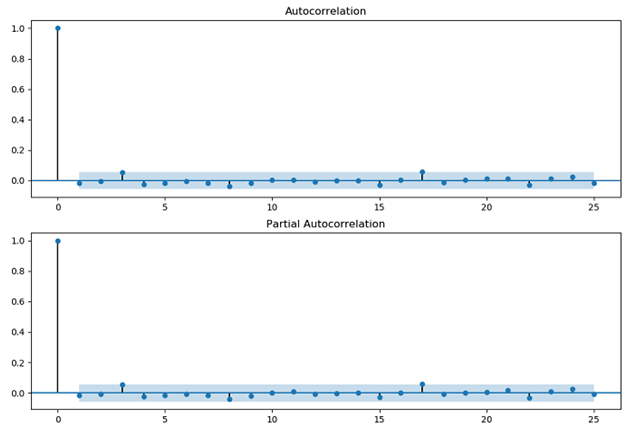It’s interesting to note that VXX returns are positively auto-correlated at 3-day lag. It’s well-known that the stock indices are mean reverting in the short term. Here we observe the opposite, i.e. a trending property.

A Volatility Trading System-Time Series Analysis in Python

Time series analysis is an important subject in finance. In this post, we are going to apply a time series technique to a financial time series and develop an investment strategy.  Specifically, we are going to use moving averages to trade volatility Exchange Traded Notes (ETN).

Moving averages are used on financial time series data to smooth out short-term noises and identify longer-term trends. We apply them to VXX, a volatility ETN. Note that VXX

• Launched in 2009
• Enables investors to bet on the size of swings in the S&P 500 Index (SPX)
• Used by individual investors and hedge fund managers alike
• Peaked at \$2 billion under management in Feb. 2018, now \$800 million
• Structured as a debt instrument with a maturity date of Jan. 30, 2019
• Was replaced by the VXXB, which has other features. Read more

The trading rules are as follows ,

If 10-day Moving Average (MA10) < 30-day Moving Average (MA30) Sell Short

If 10-day Moving Average (MA10) >= 30-day Moving Average (MA30) Cover Short

The system is implemented in Python. Graph below shows the MAs and VXX for the last 2 years.VXX and Moving Averages

The position size is \$10000; leverage is not utilized, and profit is not compounded. Graph below shows the equity curve for the trading strategy from January 2009 to January 2019.Equity curve for VXX trading system

References

 L. Connors, Buy the Fear, Sell the Greed: 7 Behavioral Quant Strategies for Traders, TradingMarkets Publishing, 2018

Post Source Here: A Volatility Trading System-Time Series Analysis in Python

Differences Between the VIX Index And At-the-Money Implied Volatility

When trading options, we often use the VIX index as a measure of volatility to help enter and manage positions. This works most of the time. However, there exist some differences between the VIX index and at-the-money implied volatility (ATM IV). In this post, we are going to show such a difference through an example. Specifically, we study the relationship between the implied volatility and forward realized volatility (RV)  of SP500. We utilize data from April 2009 to December 2018.

Recall that the VIX index

• Is a model-independent measure of volatility,
• It contains a basket of options, including out-of-the-money options. Therefore it incorporates the skew effect to some degree.

Plot below shows RV as a function of the VIX index.We observe that a high VIX index will usually lead to a higher realized volatility. The correlation between RV and the VIX is 0.6397.

For traders who manage fixed-strike options, the use of option-specific implied volatilities, in conjunction with the VIX index, should be considered. In this example, we calculate the one-month at-the-money implied volatility using SPY options. Unlike the VIX index, the fixed-strike volatilities are model-dependent. To simplify, we use the Black-Scholes model to determine the fixed-strike, fixed-maturity implied volatilities.  The constant-maturity, floating-strike implied volatilities are then calculated by interpolation.

Plot below shows RV as a function of ATM IV.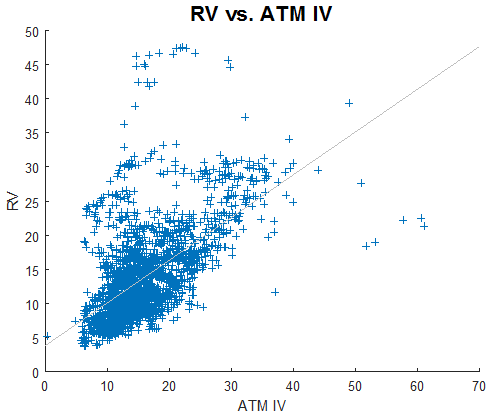We observe similar behaviour as in the previous plot. However, the correlation (0.5925) is smaller. This is probably due to the fact that ATM IV does not include the skew.

In summary:

• There are differences between the VIX index and at-the-money implied volatility.
• Higher implied volatilities (as measured by the VIX or ATM IV) will usually lead to higher RV.

Footnotes

 In this example, forward realized volatility is historical volatility shifted by one month.

Valuation of European and American Options-Derivative Pricing in Python

In previous posts, we provided examples of pricing European and American options in Excel. For pricing the European option, we utilized the Black-Scholes formula, and for pricing the American option we utilized the binomial approach. In this post, we are going to implement these methods in Python.

Recall that,

In finance, the binomial options pricing model (BOPM) provides a generalizable numerical method for the valuation of options. The binomial model was first proposed by Cox, Ross and Rubinstein in 1979. Essentially, the model uses a “discrete-time” (lattice based) model of the varying price over time of the underlying financial instrument…

The binomial pricing model traces the evolution of the option’s key underlying variables in discrete-time. This is done by means of a binomial lattice (tree), for a number of time steps between the valuation and expiration dates. Each node in the lattice represents a possible price of the underlying at a given point in time.

Valuation is performed iteratively, starting at each of the final nodes (those that may be reached at the time of expiration), and then working backwards through the tree towards the first node (valuation date). The value computed at each stage is the value of the option at that point in time. Read more

We use the same input parameters as in the previous examples.  The main input parameters are as follows,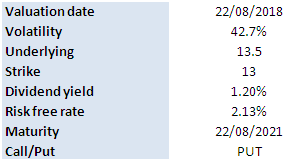Figure below shows the results calculated by the Python program. The Python program returns the same values as the Excel workbooks.Interest Rate Swap-Derivative Pricing in Python

In a previous post, we presented an example of Interest Rate Swap Pricing in Excel. In this post, we are going to provide an example of interest rate swap pricing in Python. We are going to use the USD Libor swap curve as at December 31 2018. Picture below shows the swap curve.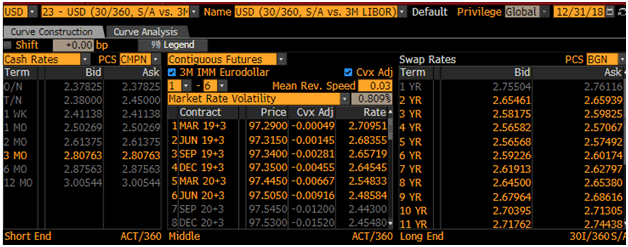USD Swap Curve as at Dec 31, 2018. Source: Bloomberg

Recall that an interest rate swap (IRS) is a financial derivative instrument that involves an exchange of a fixed interest rate for a floating interest rate.  More specifically,

An interest rate swap’s (IRS’s) effective description is a derivative contract, agreed between two counterparties, which specifies the nature of an exchange of payments benchmarked against an interest rate index. The most common IRS is a fixed for floating swap, whereby one party will make payments to the other based on an initially agreed fixed rate of interest, to receive back payments based on a floating interest rate index. Each of these series of payments is termed a ‘leg’, so a typical IRS has both a fixed and a floating leg. The floating index is commonly an interbank offered rate (IBOR) of specific tenor in the appropriate currency of the IRS, for example LIBOR in USD, GBP, EURIBOR in EUR or STIBOR in SEK. To completely determine any IRS a number of parameters must be specified for each leg; the notional principal amount (or varying notional schedule), the start and end dates and date scheduling, the fixed rate, the chosen floating interest rate index tenor, and day count conventions for interest calculations. Read more

The valuation of an interest rate swap proceeds as follows,

1. Construction of the zero-coupon curve
2. Determination of the payment schedules
3. Calculation of the net present value of future cash flows

The hypothetical interest rate swap is as follows,

Maturity: 10 years

Notional: 10 Million USD

Fixed rate: 2.5%

Floating rate:  Libor

Note that we utilize the deposit and swap rates only and ignore the futures prices in the bootstrapping process. The values of the fixed, floating legs and the interest rate swap are calculated using a Python program. We obtain the following result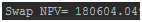Post Source Here: Interest Rate Swap-Derivative Pricing in Python

Weighted Average Cost of Capital (WACC)-Business Valuation Calculator in Excel

In this post, we are going to walk you through an example of calculating the weighted average cost of capital (WACC) using Excel.

The weighted average cost of capital (WACC) is the rate that a company is expected to pay on average to all its security holders to finance its assets. The WACC is commonly referred to as the firm’s cost of capital. Importantly, it is dictated by the external market and not by management. The WACC represents the minimum return that a company must earn on an existing asset base to satisfy its creditors, owners, and other providers of capital, or they will invest elsewhere… The WACC is calculated taking into account the relative weights of each component of the capital structure. The more complex the company’s capital structure, the more laborious it is to calculate the WACC…Companies can use WACC to see if the investment projects available to them are worthwhile to undertake. Read more

For illustration purposes, we are going to calculate the WACC of Barrick Gold, a major Canadian mining company.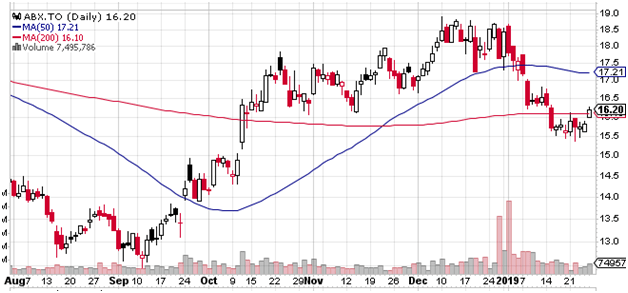Barrick Gold as at January 25 2019. Source: Stockchart.com

The WACC is calculated as follows,

WACC   =E/(E + D)*Cost of Equity + D/(E + D)*Cost of Debt*(1 – Tax Rate)

where E and D denote the market values of the firm’s equity and debt respectively. These numbers are taken from Barrick Q3 financial statement.

To calculate the cost of equity, we use the Capital Asset Pricing Model (CAPM), according to which the cost of equity is calculated as follows,

Cost of Equity = Risk-Free Rate + Beta * (Market Rate of Return – Risk-Free Rate)

Table below shows the calculation for the cost of equity as at September 30, 2018,As for the cost of debt, we will use the US Materials B- Yield Curve.  The 10-year yield is 7.651% as at the Q3 financial reporting date.

Finally, we proceed to calculate the WACC. Table below shows the calculation steps and results,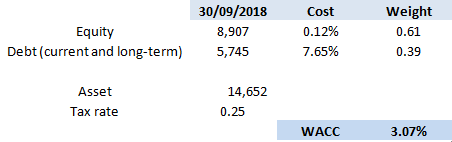Click on the link below to download the Excel workbook which contains detailed calculation steps and references.

Is Asset Dynamics Priced In Correctly by Black-Scholes-Merton Model?

A lot of research has been devoted to answering the question: do options price in the volatility risks correctly? The most noteworthy phenomenon (or bias) is called the volatility risk premium, i.e. options implied volatilities tend to overestimate future realized volatilities.  Much less attention is paid, however, to the underlying asset dynamics, i.e. to answering the question: do options price in the asset dynamics correctly?

Note that within the usual BSM framework, the underlying asset is assumed to follow a GBM process. So to answer the above question, it’d be useful to use a different process to model the asset price.

We found an interesting article on this subject .  Instead of using GBM, the authors used a process where the asset returns are auto-correlated and then developed a closed-form formula to price the options. Specifically, they assumed that the underlying asset follows an MA(1) process,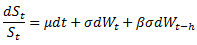where β represents the impact of past shocks and h is a small constant. We note that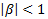and in case β=0 the price dynamics becomes GBM.

After applying some standard pricing techniques, a closed-form option pricing formula is derived which is similar to BSM except that the variance (and volatility) contains the autocorrelation coefficient,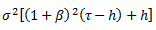From the above equation, it can be seen that

• When the underlying asset is mean reverting, i.e. β<0, which is often the case for equity indices, the MA(1) volatility becomes smaller. Therefore if we use BSM with σ as input for volatility, it will overestimate the option price.
• Conversely, when the asset is trending, i.e. β>0, BSM underestimates the option price.
• Time to maturity, τ, also affects the degree of over- underpricing. Longer-dated options will be affected more by the autocorrelation factor.

References

 Liao, S.L. and Chen, C.C. (2006), Journal of Futures Markets, 26, 85-102.

Originally Published Here: Is Asset Dynamics Priced In Correctly by Black-Scholes-Merton Model?